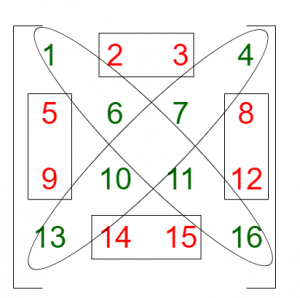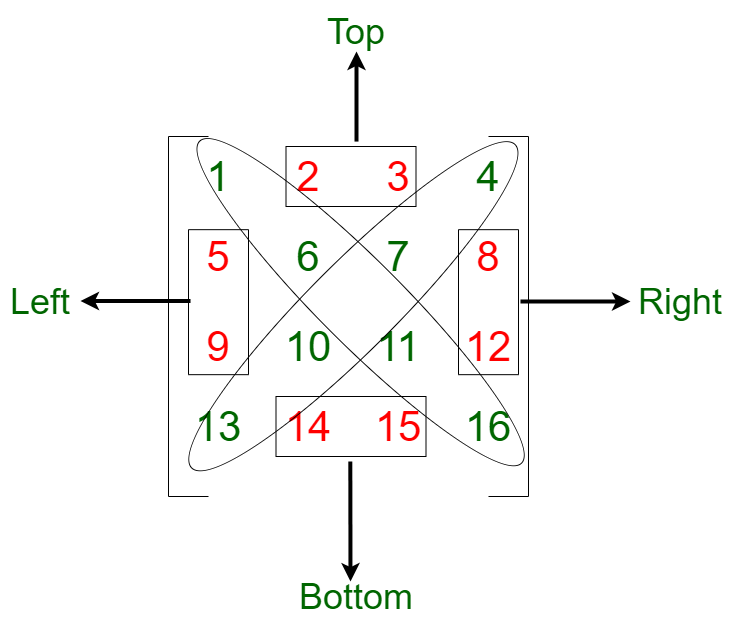# Sum of non-diagonal parts of a square Matrix

Given a square matrix of size N X N, the task is to find the sum of all elements at each portion when the matrix is divided into four parts along its diagonals. The elements at the diagonals should not be counted in the sum.

Examples:

Input: arr[][] = {{1, 2, 3, 4}, {5, 6, 7, 8}, {9, 10, 11, 12}, {13, 14, 15, 16}}
Output: 68
Explanation:From the above image, (1, 6, 11, 16) and (4, 7, 10, 13) are the diagonals.
The sum of the elements needs to be found are:
Top: (2 + 3) = 5
Left: (5 + 9) = 14
Bottom: (14 + 15) = 29
Right: (8 + 12) = 20
Therefore, sum of all parts = 68.

Input: arr[][] = { {1, 3, 1, 5}, {2, 2, 4, 1}, {5, 0, 2, 3}, {1, 3, 1, 5}}
Output: 19

## Recommended: Please try your approach on {IDE} first, before moving on to the solution.

Approach: The idea is to use indexing to identify the elements at the diagonals.

1. In a 2-dimensional matrix, two diagonals are identified in the following way:
1. Principal Diagonal: The first diagonal has the index of the row is equal to the index of the column.
```Condition for Principal Diagonal:
The row-column condition is row = column.
```
2. Secondary Diagonal: The second diagonal has the sum of the index of row and column equal to N(size of the matrix).
```Condition for Secondary Diagonal:
The row-column condition is row = numberOfRows - column -1
```
2. After identifying both the diagonals, the matrix can further be divided into two parts using the diagonal passing through the first element of the last row and the last element of the first row:
1. The left part:
• If the column index is greater than row index, the element belongs to the top portion of the matrix.
• If the row index is greater than column index, the element belongs to the left portion of the matrix.
2. The right part:
• If the column index is greater than row index, the element belongs to the right portion of the matrix.
• If the row index is greater than column index, the element belongs to the bottom portion of the matrix.3. So in order to get the sum of the non-diagonal parts of the matrix:
• Traverse the matrix rowwise
• If the element is a part of diagonal, then skip this element
• If the element is part of the left, right, bottom, or top part (i.e. non-diagonal parts), add the the element in the resultant sum

Below is the implementation of the above approach:

## C++

 `// C++ implementation of the above approach ` ` `  `#include ` `using` `namespace` `std; ` ` `  `// Function to return a vector which ` `// consists the sum of ` `// four portions of the matrix ` `int` `sumOfParts(``int``* arr, ``int` `N) ` `{ ` `    ``int` `sum_part1 = 0, sum_part2 = 0, ` `        ``sum_part3 = 0, sum_part4 = 0; ` `    ``int` `totalsum = 0; ` ` `  `    ``// Iterating through the matrix ` `    ``for` `(``int` `i = 0; i < N; i++) { ` `        ``for` `(``int` `j = 0; j < N; j++) { ` ` `  `            ``// Condition for selecting all values ` `            ``// before the second diagonal of metrics ` `            ``if` `(i + j < N - 1) { ` ` `  `                ``// Top portion of the matrix ` `                ``if` `(i < j and i != j and i + j) ` `                    ``sum_part1 += (arr + i * N)[j]; ` ` `  `                ``// Left portion of the matrix ` `                ``else` `if` `(i != j) ` `                    ``sum_part2 += (arr + i * N)[j]; ` `            ``} ` `            ``else` `{ ` ` `  `                ``// Bottom portion of the matrix ` `                ``if` `(i > j and i + j != N - 1) ` `                    ``sum_part3 += (arr + i * N)[j]; ` ` `  `                ``// Right portion of the matrix ` `                ``else` `{ ` `                    ``if` `(i + j != N - 1 and i != j) ` `                        ``sum_part4 += (arr + i * N)[j]; ` `                ``} ` `            ``} ` `        ``} ` `    ``} ` ` `  `    ``// Adding all the four portions into a vector ` `    ``totalsum = sum_part1 + sum_part2 ` `               ``+ sum_part3 + sum_part4; ` `    ``return` `totalsum; ` `} ` ` `  `// Driver code ` `int` `main() ` `{ ` `    ``int` `N = 4; ` `    ``int` `arr[N][N] = { { 1, 2, 3, 4 }, ` `                      ``{ 5, 6, 7, 8 }, ` `                      ``{ 9, 10, 11, 12 }, ` `                      ``{ 13, 14, 15, 16 } }; ` ` `  `    ``cout << sumOfParts((``int``*)arr, N); ` `} `

## Java

 `// Java implementation of the above approach ` `class` `GFG ` `{ ` `  `  `// Function to return a vector which ` `// consists the sum of ` `// four portions of the matrix ` `static` `int` `sumOfParts(``int``[][] arr, ``int` `N) ` `{ ` `    ``int` `sum_part1 = ``0``, sum_part2 = ``0``, ` `        ``sum_part3 = ``0``, sum_part4 = ``0``; ` `    ``int` `totalsum = ``0``; ` `  `  `    ``// Iterating through the matrix ` `    ``for` `(``int` `i = ``0``; i < N; i++) { ` `        ``for` `(``int` `j = ``0``; j < N; j++) { ` `  `  `            ``// Condition for selecting all values ` `            ``// before the second diagonal of metrics ` `            ``if` `(i + j < N - ``1``) { ` `  `  `                ``// Top portion of the matrix ` `                ``if` `(i < j && i != j && i + j > ``0``) ` `                    ``sum_part1 += arr[i][j]; ` `  `  `                ``// Left portion of the matrix ` `                ``else` `if` `(i != j) ` `                    ``sum_part2 += arr[i][j]; ` `            ``} ` `            ``else` `{ ` `  `  `                ``// Bottom portion of the matrix ` `                ``if` `(i > j && i + j != N - ``1``) ` `                    ``sum_part3 += arr[i][j]; ` `  `  `                ``// Right portion of the matrix ` `                ``else` `{ ` `                    ``if` `(i + j != N - ``1` `&& i != j) ` `                        ``sum_part4 += arr[i][j]; ` `                ``} ` `            ``} ` `        ``} ` `    ``} ` `  `  `    ``// Adding all the four portions into a vector ` `    ``totalsum = sum_part1 + sum_part2 ` `               ``+ sum_part3 + sum_part4; ` `    ``return` `totalsum; ` `} ` `  `  `// Driver code ` `public` `static` `void` `main(String[] args) ` `{ ` `    ``int` `N = ``4``; ` `    ``int` `arr[][] = { { ``1``, ``2``, ``3``, ``4` `}, ` `                      ``{ ``5``, ``6``, ``7``, ``8` `}, ` `                      ``{ ``9``, ``10``, ``11``, ``12` `}, ` `                      ``{ ``13``, ``14``, ``15``, ``16` `} }; ` `  `  `    ``System.out.print(sumOfParts(arr, N)); ` `} ` `} ` ` `  `// This code is contributed by PrinciRaj1992 `

## Python3

 `# Python3 implementation of the above approach ` ` `  `# Function to return a vector which ` `# consists the sum of ` `# four portions of the matrix ` `def` `sumOfParts(arr,N): ` `    ``sum_part1, sum_part2, sum_part3, \ ` `    ``sum_part4 ``=` `0``, ``0``, ``0``, ``0` `    ``totalsum ``=` `0` ` `  `    ``# Iterating through the matrix ` `    ``for` `i ``in` `range``(N): ` `        ``for` `j ``in` `range``(N): ` `             `  `            ``# Condition for selecting all values ` `            ``# before the second diagonal of metrics ` `            ``if` `i ``+` `j < N ``-` `1``: ` `                 `  `                ``# Top portion of the matrix ` `                ``if``(i < j ``and` `i !``=` `j ``and` `i ``+` `j): ` `                    ``sum_part1 ``+``=` `arr[i][j] ` `                 `  `                ``# Left portion of the matrix ` `                ``elif` `i !``=` `j: ` `                    ``sum_part2 ``+``=` `arr[i][j] ` `            ``else``: ` `                 `  `                ``# Bottom portion of the matrix ` `                ``if` `i > j ``and` `i ``+` `j !``=` `N ``-` `1``: ` `                    ``sum_part3 ``+``=` `arr[i][j] ` `                ``else``: ` `                     `  `                ``# Right portion of the matrix ` `                    ``if` `i ``+` `j !``=` `N ``-` `1` `and` `i !``=` `j: ` `                        ``sum_part4 ``+``=` `arr[i][j] ` `        ``# Adding all the four portions into a vecto ` `    ``return` `sum_part1 ``+` `sum_part2 ``+` `sum_part3 ``+` `sum_part4 ` ` `  `# Driver code ` `N ``=` `4` `arr ``=` `[[ ``1``, ``2``, ``3``, ``4` `], ` `       ``[ ``5``, ``6``, ``7``, ``8` `], ` `       ``[ ``9``, ``10``, ``11``, ``12` `], ` `       ``[ ``13``, ``14``, ``15``, ``16` `]] ` ` `  `print``(sumOfParts(arr, N)) ` ` `  `# This code is contributed by mohit kumar 29 `

## C#

 `// C# implementation of the above approach ` `using` `System; ` ` `  `class` `GFG ` `{ ` `  `  `    ``// Function to return a vector which ` `    ``// consists the sum of ` `    ``// four portions of the matrix ` `    ``static` `int` `sumOfParts(``int``[,] arr, ``int` `N) ` `    ``{ ` `        ``int` `sum_part1 = 0, sum_part2 = 0, ` `            ``sum_part3 = 0, sum_part4 = 0; ` `        ``int` `totalsum = 0; ` `      `  `        ``// Iterating through the matrix ` `        ``for` `(``int` `i = 0; i < N; i++) { ` `            ``for` `(``int` `j = 0; j < N; j++) { ` `      `  `                ``// Condition for selecting all values ` `                ``// before the second diagonal of metrics ` `                ``if` `(i + j < N - 1) { ` `      `  `                    ``// Top portion of the matrix ` `                    ``if` `(i < j && i != j && i + j > 0) ` `                        ``sum_part1 += arr[i, j]; ` `      `  `                    ``// Left portion of the matrix ` `                    ``else` `if` `(i != j) ` `                        ``sum_part2 += arr[i, j]; ` `                ``} ` `                ``else` `{ ` `      `  `                    ``// Bottom portion of the matrix ` `                    ``if` `(i > j && i + j != N - 1) ` `                        ``sum_part3 += arr[i, j]; ` `      `  `                    ``// Right portion of the matrix ` `                    ``else` `{ ` `                        ``if` `(i + j != N - 1 && i != j) ` `                            ``sum_part4 += arr[i, j]; ` `                    ``} ` `                ``} ` `            ``} ` `        ``} ` `      `  `        ``// Adding all the four portions into a vector ` `        ``totalsum = sum_part1 + sum_part2 ` `                   ``+ sum_part3 + sum_part4; ` `        ``return` `totalsum; ` `    ``} ` `      `  `    ``// Driver code ` `    ``public` `static` `void` `Main() ` `    ``{ ` `        ``int` `N = 4; ` `        ``int` `[,]arr = { { 1, 2, 3, 4 }, ` `                          ``{ 5, 6, 7, 8 }, ` `                          ``{ 9, 10, 11, 12 }, ` `                          ``{ 13, 14, 15, 16 } }; ` `      `  `        ``Console.WriteLine(sumOfParts(arr, N)); ` `    ``} ` `} ` ` `  `// This code is contributed by Yash_R `

Output:

```68
```

Time Complexity: O(N2) as we are traversing the complete matrix rowwise.

Attention reader! Don’t stop learning now. Get hold of all the important DSA concepts with the DSA Self Paced Course at a student-friendly price and become industry ready.

My Personal Notes arrow_drop_upCheck out this Author's contributed articles.

If you like GeeksforGeeks and would like to contribute, you can also write an article using contribute.geeksforgeeks.org or mail your article to contribute@geeksforgeeks.org. See your article appearing on the GeeksforGeeks main page and help other Geeks.

Please Improve this article if you find anything incorrect by clicking on the "Improve Article" button below.